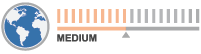# Windows 8 Date Calculation

Time is a very important factor within our everyday lives. We frequently formulate agendas to achieve as many things as possible, whilst wasting the least amount of time. This tutorial is useful for people, especially those in business, who need to determine estimated times and dates. This guide explains how to calculate the number of days between two dates (the interval), and how to determine an exact date after adding or subtracting a specific period of time. For example, if you need to visit the doctor in say five weeks, it would be easier to use a calculator rather than counting the days manually on a calendar. Many computer users do not realize that Windows 8 (as well as Windows 7) has a calculator with a built-in date calculation function.

Note: when you use the date calculator, the standard calculator is not functional. To revert, left-click on the mouse.

1. Open the calculator. If you cannot find it, type 'calculator' in the Start menu and the search result will appear automatically.2. Next, press View>Date calculation (or Ctrl+E) to change the type of calculator.3. There are two methods of calculation: i) addition/subtraction, and; ii) calculating the difference between two dates.4. To calculate the difference, choose two dates and click 'Calculate'.5. To add days, change the type of calculation (as shown in step 3), and then choose the date and the amount of time you wish to add.6. To subtract, check the 'Subtract' option beside the date.7. To revert the calculator to the standard type, click View>Basic (or Ctrl+F4).Using date calculation, you can regulate your time and determine the exact dates after a specific number of months, days, etc., in a straightforward and easy-to-use way. Windows 8 employs some great built-in features such as this to make PC users' lives easier.

Here is a video showing how to use date calculation in Windows 8:

▼ Show Discussion